Documentation

### This is machine translation

Mouseover text to see original. Click the button below to return to the English version of the page.

To view all translated materials including this page, select Country from the country navigator on the bottom of this page.

# bode

Bode plot of frequency response, or magnitude and phase data

## Syntax

``bode(sys)``
``bode(sys1,sys2,...,sysN)``
``bode(sys1,LineSpec1,...,sysN,LineSpecN)``
``bode(___,w)``
``````[mag,phase,wout] = bode(sys)``````
``````[mag,phase,wout] = bode(sys,w)``````
``````[mag,phase,wout,sdmag,sdphase] = bode(sys,w)``````

## Description

example

````bode(sys)` creates a Bode plot of the frequency response of a dynamic system model `sys`. The plot displays the magnitude (in dB) and phase (in degrees) of the system response as a function of frequency. `bode` automatically determines frequencies to plot based on system dynamics. If `sys` is a multi-input, multi-output (MIMO) model, then `bode` produces an array of Bode plots, each plot showing the frequency response of one I/O pair. ```

example

````bode(sys1,sys2,...,sysN)` plots the frequency response of multiple dynamic systems on the same plot. All systems must have the same number of inputs and outputs.```

example

````bode(sys1,LineSpec1,...,sysN,LineSpecN)` specifies a color, linestyle, and marker for each system in the plot.```

example

````bode(___,w)` plots system responses for frequencies specified by `w`.If `w` is a cell array of the form `{wmin,wmax}`, then `bode` plots the response at frequencies ranging between `wmin` and `wmax`.If `w` is a vector of frequencies, then `bode` plots the response at each specified frequency.You can use `w` with any of the input-argument combinations in previous syntaxes.```

example

``````[mag,phase,wout] = bode(sys)``` returns the magnitude and phase of the response at each frequency in the vector `wout`. The function automatically determines frequencies in `wout` based on system dynamics. This syntax does not draw a plot.```

example

``````[mag,phase,wout] = bode(sys,w)``` returns the response data at the frequencies specified by `w`.If `w` is a cell array of the form `{wmin,wmax}`, then `wout` contains frequencies ranging between `wmin` and `wmax`.If `w` is a vector of frequencies, then `wout` = `w`. ```

example

``````[mag,phase,wout,sdmag,sdphase] = bode(sys,w)``` also returns the estimated standard deviation of the magnitude and phase values for the identified model `sys`. If you omit `w`, then the function automatically determines frequencies in `wout` based on system dynamics.```

## Examples

collapse all

Create a Bode plot of the following continuous-time SISO dynamic system.

`$H\left(s\right)=\frac{{s}^{2}+0.1s+7.5}{{s}^{4}+0.12{s}^{3}+9{s}^{2}}.$`

```H = tf([1 0.1 7.5],[1 0.12 9 0 0]); bode(H)```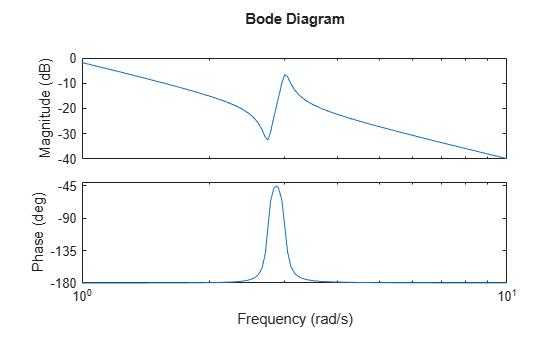`bode` automatically selects the plot range based on the system dynamics.

Create a Bode plot over a specified frequency range. Use this approach when you want to focus on the dynamics in a particular range of frequencies.

```H = tf([-0.1,-2.4,-181,-1950],[1,3.3,990,2600]); bode(H,{1,100}) grid on```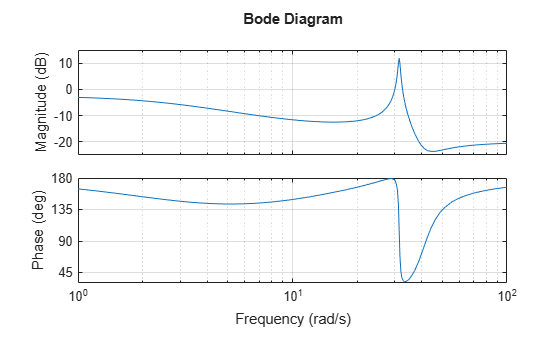The cell array `{1,100}` specifies the minimum and maximum frequency values in the Bode plot. When you provide frequency bounds in this way, the function selects intermediate points for frequency response data.

Alternatively, specify a vector of frequency points to use for evaluating and plotting the frequency response.

```w = [1 5 10 15 20 23 31 40 44 50 85 100]; bode(H,w,'.-') grid on```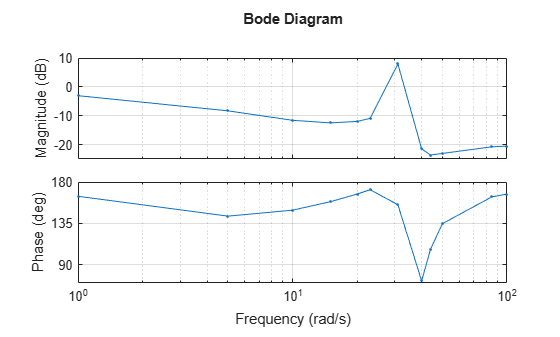`bode` plots the frequency response at the specified frequencies only.

Compare the frequency response of a continuous-time system to an equivalent discretized system on the same Bode plot.

Create continuous-time and discrete-time dynamic systems.

```H = tf([1 0.1 7.5],[1 0.12 9 0 0]); Hd = c2d(H,0.5,'zoh');```

Create a Bode plot that displays both systems.

`bode(H,Hd)`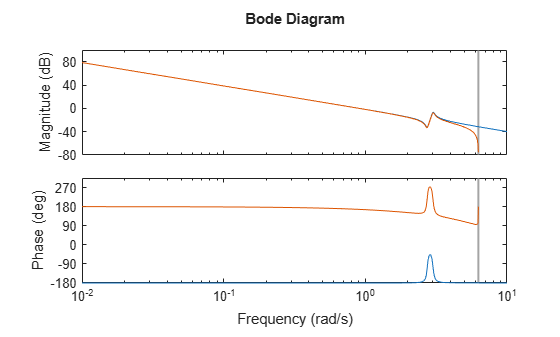The Bode plot of a discrete-time system includes a vertical line marking the Nyquist frequency of the system.

Specify the line style, color, or marker for each system in a Bode plot using the `LineSpec` input argument.

```H = tf([1 0.1 7.5],[1 0.12 9 0 0]); Hd = c2d(H,0.5,'zoh'); bode(H,'r',Hd,'b--')```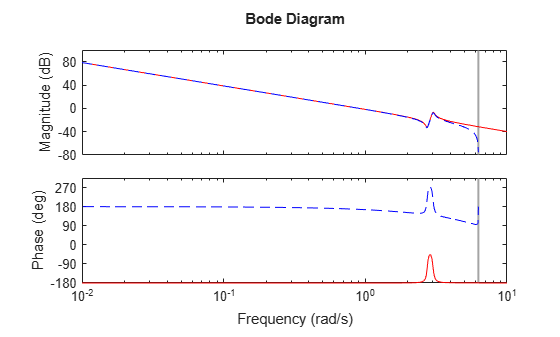The first `LineSpec`, `'r'`, specifies a solid red line for the response of `H`. The second `LineSpec`, `'b--'`, specifies a dashed blue line for the response of `Hd`.

Compute the magnitude and phase of the frequency response of a SISO system.

If you do not specify frequencies, `bode` chooses frequencies based on the system dynamics and returns them in the third output argument.

```H = tf([1 0.1 7.5],[1 0.12 9 0 0]); [mag,phase,wout] = bode(H);```

Because `H` is a SISO model, the first two dimensions of `mag` and `phase` are both 1. The third dimension is the number of frequencies in `wout`.

`size(mag)`
```ans = 1×3 1 1 42 ```
`length(wout)`
```ans = 42 ```

Thus, each entry along the third dimension of `mag` gives the magnitude of the response at the corresponding frequency in `wout`.

For this example, create a 2-output, 3-input system.

```rng(0,'twister'); % For reproducibility H = rss(4,2,3);```

For this system, `bode` plots the frequency responses of each I/O channel in a separate plot in a single figure.

`bode(H)`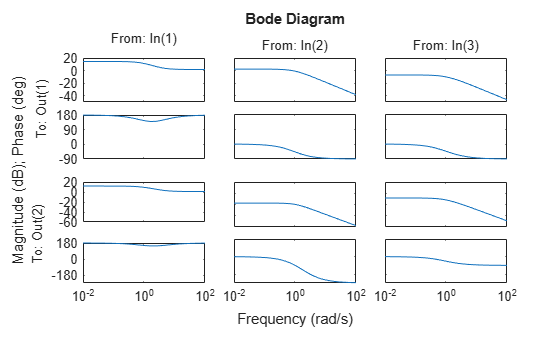Compute the magnitude and phase of these responses at 20 frequencies between 1 and 10 radians.

```w = logspace(0,1,20); [mag,phase] = bode(H,w);```

`mag` and `phase` are three-dimensional arrays, in which the first two dimensions correspond to the output and input dimensions of `H`, and the third dimension is the number of frequencies. For instance, examine the dimensions of `mag`.

`size(mag)`
```ans = 1×3 2 3 20 ```

Thus, for example, `mag(1,3,10)` is the magnitude of the response from the third input to the first output, computed at the 10th frequency in `w`. Similarly, `phase(1,3,10)` contains the phase of the same response.

Compare the frequency response of a parametric model, identified from input/output data, to a nonparametric model identified using the same data.

Identify parametric and nonparametric models based on data.

```load iddata2 z2; w = linspace(0,10*pi,128); sys_np = spa(z2,[],w); sys_p = tfest(z2,2);```

Using the `spa` and `tfest` commands requires System Identification Toolbox™ software.

`sys_np` is a nonparametric identified model. `sys_p` is a parametric identified model.

Create a Bode plot that includes both systems.

```bode(sys_np,sys_p,w); legend('sys-np','sys-p')```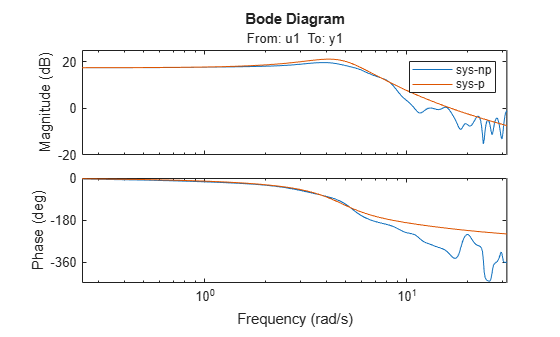You can display the confidence region on the Bode plot by right-clicking the plot and selecting Characteristics > Confidence Region.

Compute the standard deviation of the magnitude and phase of an identified model. Use this data to create a 3σ plot of the response uncertainty.

Identify a transfer function model based on data. Obtain the standard deviation data for the magnitude and phase of the frequency response.

```load iddata2 z2; sys_p = tfest(z2,2); w = linspace(0,10*pi,128); [mag,ph,w,sdmag,sdphase] = bode(sys_p,w);```

Using the `tfest` command requires System Identification Toolbox™ software.

`sys_p` is an identified transfer function model. `sdmag` and `sdphase` contain the standard deviation data for the magnitude and phase of the frequency response, respectively.

Use the standard deviation data to create a 3σ plot corresponding to the confidence region.

```mag = squeeze(mag); sdmag = squeeze(sdmag); semilogx(w,mag,'b',w,mag+3*sdmag,'k:',w,mag-3*sdmag,'k:');```## Input Arguments

collapse all

Dynamic system, specified as a SISO or MIMO dynamic system model or array of dynamic system models. Dynamic systems that you can use include:

• Continuous-time or discrete-time numeric LTI models, such as `tf`, `zpk`, or `ss` models.

• Generalized or uncertain LTI models such as `genss` or `uss` models. (Using uncertain models requires Robust Control Toolbox™ software.)

• For tunable control design blocks, the function evaluates the model at its current value for both plotting and returning frequency response data.

• For uncertain control design blocks, the function plots the nominal value and random samples of the model. When you use output arguments, the function returns frequency response data for the nominal model only.

• Frequency-response data models such as `frd` models. For such models, the function plots the response at frequencies defined in the model.

• Identified LTI models, such as `idtf`, `idss`, or `idproc` models. For such models, the function can also plot confidence intervals and return standard deviations of the frequency response. See Bode Plot of Identified Model. (Using identified models requires System Identification Toolbox™ software.)

If `sys` is an array of models, the function plots the frequency responses of all models in the array on the same axes.

Line style, marker, and color, specified as a string or vector of one, two, or three characters. The characters can appear in any order. You do not need to specify all three characteristics (line style, marker, and color). For example, if you omit the line style and specify the marker, then the plot shows only the marker and no line. For more information about configuring this argument, see the `LineSpec` input argument of the `plot` function.

Example: `'r--'` specifies a red dashed line

Example: `'*b'` specifies blue asterisk markers

Example: `'y'` specifies a yellow line

Frequencies at which to compute and plot frequency response, specified as the cell array `{wmin,wmax}` or as a vector of frequency values.

• If `w` is a cell array of the form `{wmin,wmax}`, then the function computes the index at frequencies ranging between `wmin` and `wmax`.

• If `w` is a vector of frequencies, then the function computes the index at each specified frequency. For example, use `logspace` to generate a row vector with logarithmically spaced frequency values.

Specify frequencies in units of rad/`TimeUnit`, where `TimeUnit` is the `TimeUnit` property of the model.

## Output Arguments

collapse all

Magnitude of the system response in absolute units, returned as a 3-D array. The dimensions of this array are (number of system outputs) × (number of system inputs) × (number of frequency points).

To convert the magnitude from absolute units to decibels, use:

```magdb = 20*log10(mag) ```

Phase of the system response in degrees, returned as a 3-D array. The dimensions of this array are (number of outputs) × (number of inputs) × (number of frequency points).

Frequencies at which the function returns the system response, returned as a column vector. The function chooses the frequency values based on the model dynamics, unless you specify frequencies using the input argument `w`.

Frequency values are in radians per `TimeUnit`, where `TimeUnit` is the value of the `TimeUnit` property of `sys`.

Estimated standard deviation of the magnitude of the response at each frequency point, returned as a 3-D array. `sdmag` has the same dimensions as `mag`.

If `sys` is not an identified LTI model, `sdmag` is `[]`.

Estimated standard deviation of the phase of the response at each frequency point, returned as a 3-D array. `sdphase` has the same dimensions as `phase`.

If `sys` is not an identified LTI model, `sdphase` is `[]`.

## Algorithms

`bode` computes the frequency response as follows:

1. Compute the zero-pole-gain (`zpk`) representation of the dynamic system.

2. Evaluate the gain and phase of the frequency response based on the zero, pole, and gain data for each input/output channel of the system.

• For continuous-time systems, `bode` evaluates the frequency response on the imaginary axis s = and considers only positive frequencies.

• For discrete-time systems, `bode` evaluates the frequency response on the unit circle. To facilitate interpretation, the command parameterizes the upper half of the unit circle as:

`$z={e}^{j\omega {T}_{s}},\text{ }0\le \omega \le {\omega }_{N}=\frac{\pi }{{T}_{s}},$`

where Ts is the sample time and ωN is the Nyquist frequency. The equivalent continuous-time frequency ω is then used as the x-axis variable. Because $H\left({e}^{j\omega {T}_{s}}\right)$ is periodic with period 2ωN, `bode` plots the response only up to the Nyquist frequency ωN. If `sys` is a discrete-time model with unspecified sample time, `bode` uses Ts = 1.